• Slides: 13The Quadratic Formula For any quadratic equation of the form The solutions are given by the formula:Example Solve 3 x 2 + 5 x = 2 using the quadratic formula. Solution First determine a, b, and c: 3 x 2 + 5 x – 2 = 0; a = 3, b = 5, and c = – 2. Substituting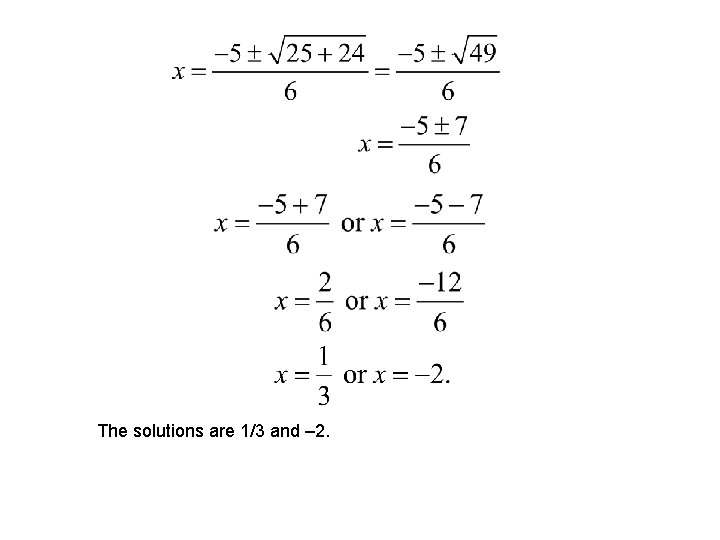The solutions are 1/3 and – 2.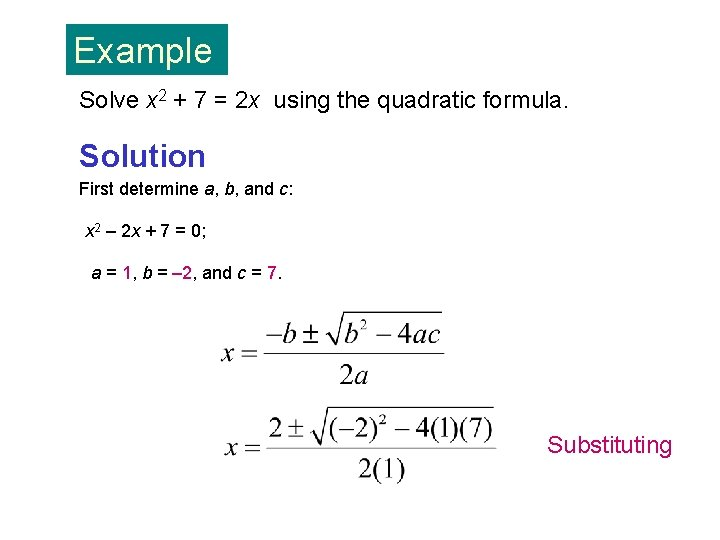Example Solve x 2 + 7 = 2 x using the quadratic formula. Solution First determine a, b, and c: x 2 – 2 x + 7 = 0; a = 1, b = – 2, and c = 7. SubstitutingThe solutions areExamples Solve the following quadratic equations: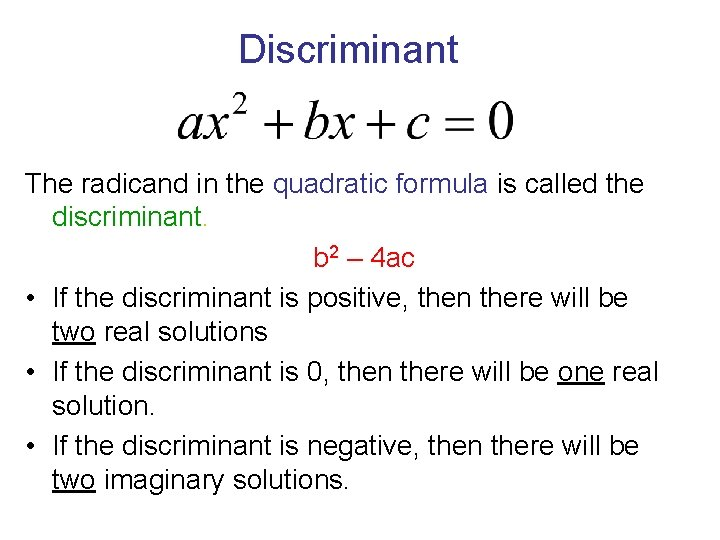Discriminant The radicand in the quadratic formula is called the discriminant. b 2 – 4 ac • If the discriminant is positive, then there will be two real solutions • If the discriminant is 0, then there will be one real solution. • If the discriminant is negative, then there will be two imaginary solutions.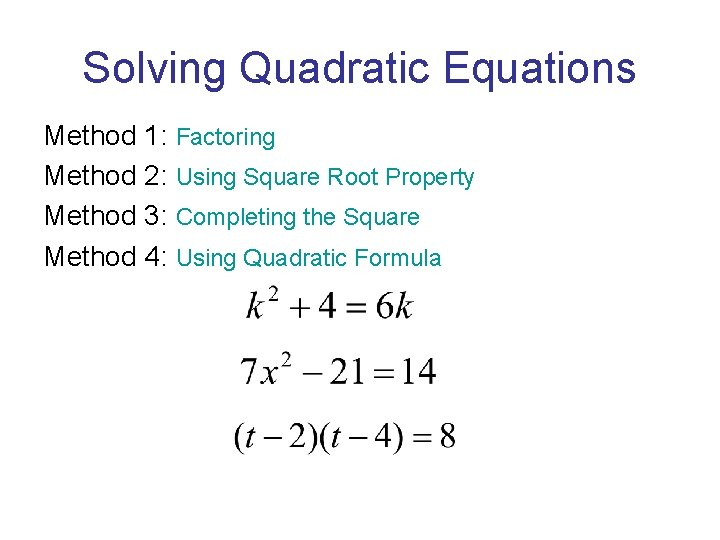Solving Quadratic Equations Method 1: Factoring Method 2: Using Square Root Property Method 3: Completing the Square Method 4: Using Quadratic Formula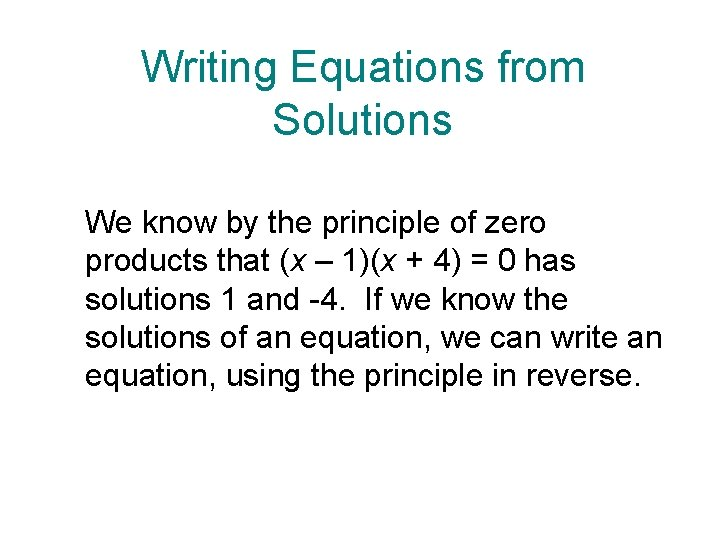Writing Equations from Solutions We know by the principle of zero products that (x – 1)(x + 4) = 0 has solutions 1 and -4. If we know the solutions of an equation, we can write an equation, using the principle in reverse.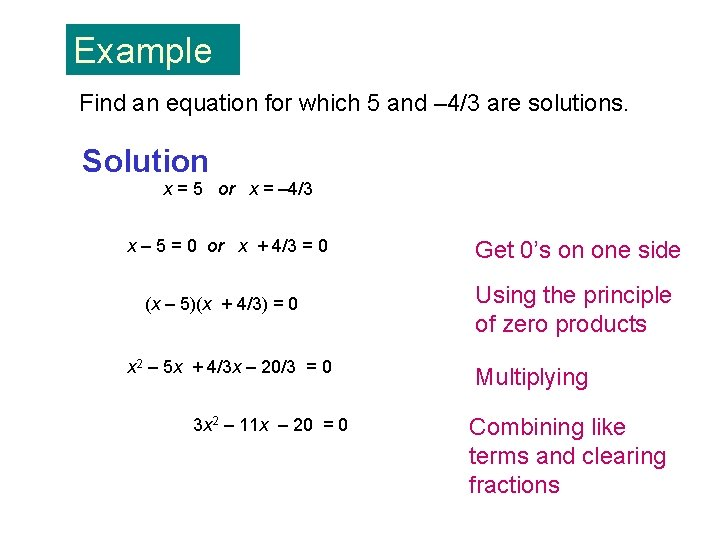Example Find an equation for which 5 and – 4/3 are solutions. Solution x = 5 or x = – 4/3 x – 5 = 0 or x + 4/3 = 0 (x – 5)(x + 4/3) = 0 x 2 – 5 x + 4/3 x – 20/3 = 0 3 x 2 – 11 x – 20 = 0 Get 0’s on one side Using the principle of zero products Multiplying Combining like terms and clearing fractions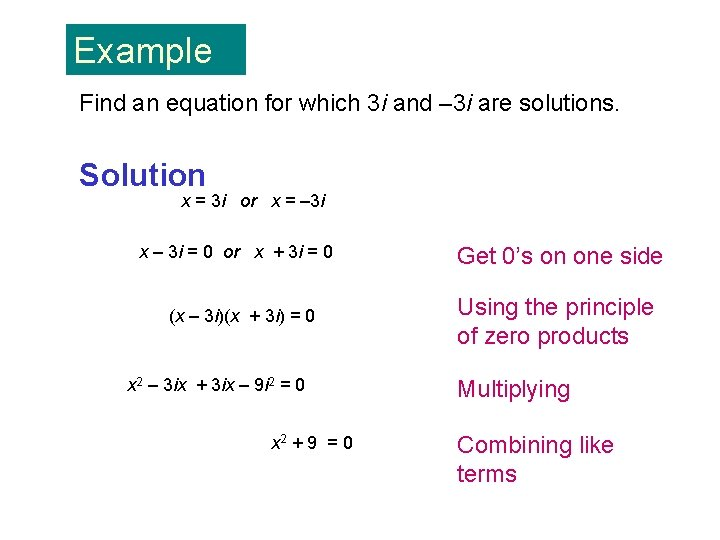Example Find an equation for which 3 i and – 3 i are solutions. Solution x = 3 i or x = – 3 i x – 3 i = 0 or x + 3 i = 0 (x – 3 i)(x + 3 i) = 0 x 2 – 3 ix + 3 ix – 9 i 2 = 0 x 2 + 9 = 0 Get 0’s on one side Using the principle of zero products Multiplying Combining like termsExample The braking distance, d (in feet), of a car going v miles per hour is given by How fast would a car be traveling if its braking distance is 150 ft?